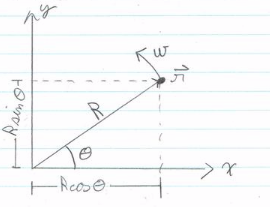### Solution Found!# A particle moves in a circle (center 0 and radius R) with

Chapter 1, Problem 1.1

(choose chapter or problem)

Get Unlimited Answers! Check out our subscriptions
QUESTION:

A particle moves in a circle (center O and radius R) with constant angular velocity $$\omega$$ counter-clockwise. The circle lies in the xy plane and the particle is on the x axis at time t = 0. Show that the particle's position is given by

$$\mathrm{r}(t)=\hat{\mathrm{x}} R \cos (\omega t)+\hat{\mathrm{y}} R \sin (\omega t)$$

Find the particle's velocity and acceleration. What are the magnitude and direction of the acceleration? Relate your results to well-known properties of uniform circular motion.Want To Learn More? To watch the entire video and ALL of the videos in the series:

QUESTION:

A particle moves in a circle (center O and radius R) with constant angular velocity $$\omega$$ counter-clockwise. The circle lies in the xy plane and the particle is on the x axis at time t = 0. Show that the particle's position is given by

$$\mathrm{r}(t)=\hat{\mathrm{x}} R \cos (\omega t)+\hat{\mathrm{y}} R \sin (\omega t)$$

Find the particle's velocity and acceleration. What are the magnitude and direction of the acceleration? Relate your results to well-known properties of uniform circular motion.

Step 1 of 4

Let's find the $$x$$ and $$y$$ components of the position vector $$r$$ with trigonometry.Also and we know that $$\theta=\omega t$$

without loss of generality we can set the phase to 0 , which means $$\theta=\omega t$$ without adding a phase shift.

$$\begin{array}{l} \vec{r}_{x}=R \cos \theta \text { and } \vec{r}_{y}=R \sin \theta \\ \Rightarrow \vec{r}(t)=R \cos (\omega t) \hat{\mathbf{x}}+R \sin (\omega t) \hat{\mathbf{y}} \end{array}$$